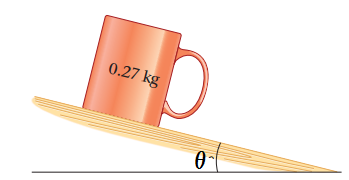# Problem: A mug rests on an inclined surface, as shown in (Figure 1) , θ=10∘.A.) What is the magnitude of the frictional force exerted on the mug?B.) What is the minimum coefficient of static friction required to keep the mug from sliding?

###### FREE Expert Solution

Newton's second law:

$\overline{){\mathbf{\Sigma }}{\mathbf{F}}{\mathbf{=}}{\mathbf{m}}{\mathbf{a}}}$

83% (228 ratings)###### Problem Details

A mug rests on an inclined surface, as shown in (Figure 1) , θ=10∘.A.) What is the magnitude of the frictional force exerted on the mug?
B.) What is the minimum coefficient of static friction required to keep the mug from sliding?

Frequently Asked Questions

What scientific concept do you need to know in order to solve this problem?

Our tutors have indicated that to solve this problem you will need to apply the Inclined Planes with Friction concept. You can view video lessons to learn Inclined Planes with Friction. Or if you need more Inclined Planes with Friction practice, you can also practice Inclined Planes with Friction practice problems.

What professor is this problem relevant for?

Based on our data, we think this problem is relevant for Professor Hearn's class at NMSU.